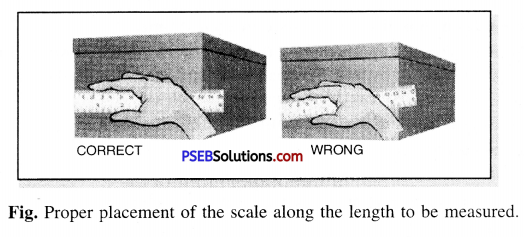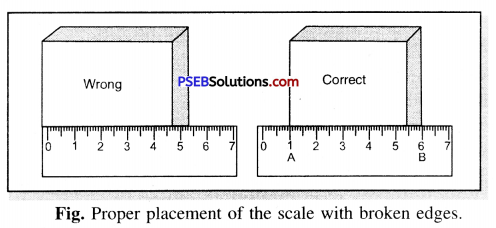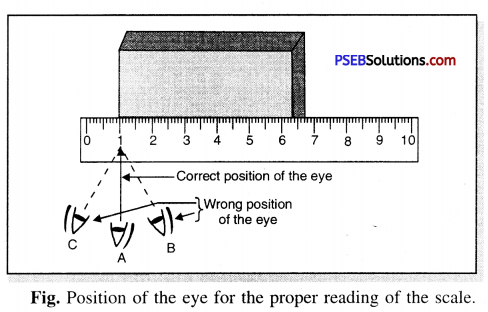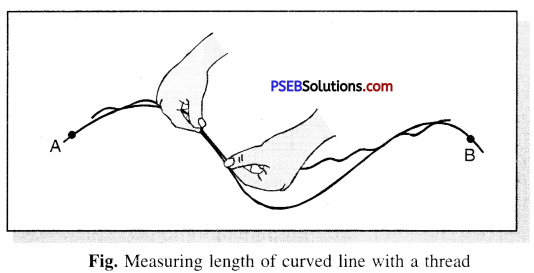# PSEB 6th Class Science Solutions Chapter 10 Motion and Measurement of Distances

Punjab State Board PSEB 6th Class Science Book Solutions Chapter 10 Motion and Measurement of Distances Textbook Exercise Questions and Answers.

## PSEB Solutions for Class 6 Science Chapter 10 Motion and Measurement of Distances

Science Guide for Class 6 PSEB Motion and Measurement of Distances Intext Questions and Answers

Think and Answer (Textbook Page No. 103)

Question 1.
The length of a curved line can be measured by a metre scale directly. (True/False)
False.

Question 2.
The metre is standard unit of length. (True/False)
True.

PSEB 6th Class Science Guide Motion and Measurement of Distances Textbook Questions and Answers

Exercise – 1

1. Fill in the Blanks :

(a) One metre is ………………… cm.
100(b) Five kilometre is ………………. m.
5000

(c) Motion of a child on a swing is ……………….
Periodic motion

(d) Motion of the needle of a sewing machine is ……………….
Periodic motion

(e) Motion of wheel of a bicycle is ……………..
Circular motion

2. Write True or False:

(a) Handspan or foot step are standard units of measurement.
False

(b) Standard unit of length is metre.
True

(c) Motion of train on railway track is an example of linear motion.
True(d) Length of a curved line can be measured by a scale.
False

(e) Motion of hands of a clock is a circular motion.
True

3. Match the Column A with Column B:

 Column A Column B 1. Metre (a) Periodic Motion 2. Circular Motion (b) Length 3. 1 Km (c) Linear Motion 4. Train on track (d) Hands of Clock 5. Swing (e) 1000 m

 Column A Column B 1. Metre (b) Length 2. Circular Motion (d) Hands of Clock 3. 1 Km (e) 1000 m 4. Train on track (c) Linear Motion 5. Swing (a) Periodic Motion

Exercise – 2

Question (i)
In motor vehicles which of the following is used for measuring the distance covered by the vehicle ?
(a) Speedometer
(b) Odometer
(c) Thermometer
(d) None of these.
(b) Odometer

Question (ii)
A student want to take the measurements of a brick, for this purpose which unit he should use ?
(a) Kilometre
(b) Meter
(c) Centimetre
(d) Hand span.
(c) Centimetre

Question (iii)
Which one among the following is false ?
(a) 1000 m = 1 km
(b) 100 mm = 1 cm
(c) 100 cm = 1 m
(d) 10 mm = 1 cm.
(b) 100 mm = 1 cmQuestion (iv)
The motion of wings of a moving fan is …………….
(a) Circular Motion
(b) Linear Motion
(c) Both (a) and (b)
(d) None of these.
(a) Circular Motion.

5. Very Short Answer Type Questions:

Question (i)
Name some means of transport used in ancient time.
Ancient means of transport :

1. Bullock Cart,
2. Hand drawn and hand pushed carts,
3. Palanquin carried by men,
5. walked on foot,
6. rode on animals back (horse and donkey),
7. Tonga, (8) Coracles.

Question (ii)
Give two examples of periodic motion.
Examples of Periodic motion :
(1) Motion of simple pendulum
(2) Up and down motion of needle of sewing machine.
(3) Motion of swing.

Question (i)
Give two examples of transport used on land, water and air.
Examples of transport :
(1) On land : (a) Bus, (b) Car, (c) Train.
(2) On Water : (a) Ship, (b) Boat.
(3) In Air : (a) Aeroplane, (b) Helicopter.

Question (ii)
What is distance ?
Distance. It is measurement of length between two points. It is also a length and S.l. unit of distance is metre.

Question (iii)
Arrange the following lengths in their incresing magnitude :
1 metre, 1 centimetre, 1 kilometre, 1 millimetre.
Arrangement of lengths in increasing magnitude :
1 millimeter < 1 centimeter < 1 metre < 1 kilometer.

Question (iv)
Write the similarities and differences between the motion of a bicycle and a ceiling fan that has been switched on.
Both bicycle and ceiling fan have circular motion. But wheel of a bi-cycle also performs linear motion on the road which is not undertaken by ceiling fan.Question (v)
The distance between Radha’s home and her school is 3250 m. Express this distance into km.
Solution.
Distance between Radha’s home and school = 3250 m
= $$\frac{3250}{1000}$$ km [∵ 1 km – 1000 m]
= 3.250 km

7. Long Answer Type Questions :

Question (i)
Describe what precautions should be taken while measuring length or breadth of an object.
The following precautions must be taken while using a metre scale for measuring length :
(1) The scale should be placed close and along the length of the object to be measured.(2) If the ends of the scale are broken then in such a case one should coincide any other full mark of the scale with one edge of the object. The correct length is obtained by subtracting the reading of the mark from the reading coinciding with the other end of the object.(3) The eye must be kept on the end point of the object where the measurement is to be taken.Question (ii)
How many types of motion are there ? Give examples for each type.
Different types of motion that we generally observe in our daily life are given below :
1. Linear Motion,
2. Circular Motion,
3. Periodic Motion.

1. Linear Motion. The motion of an object in a straight line is called Linear Motion.
Examples :
(a) A car or bus moving on a straight road.
(b) An athlete running on a straight track.
(c) Train running on straight track.

2. Circular Motion. Motion of an object on a circular path is called circular motion.
Examples :
(a) Motion of blades of a ceiling fan.
(b) Motion of hands of a wall clock.
(c) Motion of stone tied to the string when whirled around.

3. Periodic Motion. When an object repeats its motion after a fixed interval of time is said to have Periodic Motion.
Examples :
(a) The motion of a swing.
(b) Motion of needle of a sewing machine.
(c) Heart beat.
(d) The revolution of earth around the sun.

Question (iii)
Write an activity for measuring the length of a curved line.
Measuring length of curved line using a thread :
The following steps should be taken while measuring the length of a curved line :
(1) Take a long, thick piece of thread.
(2) Tie a knot at one end of the thread.
(3) Place this knot on one point of curve, let it be A.(4) Place small portion of thread along the line using fingers and thumb.
(5) Hold the thread at this end point with one hand.
(6) Using other hand, stretch a little more portion of thread along the curved line.
(7) Repeat this process till you reach the other end of line, let it be point B,
(8) Mark the point on the thread with ink.
(9) Stretch this thread on a metre scale and measure the length between the knot and the end mark on the thread. This is the length of the curved line.Question (iv)
Why handspan or foot steps cannot be used as standard units of measurement ?
A standard unit must be same at all places so that it is understood similarly by different persons. If a length is measured by handspans or foot steps of different person it will not be the same.
As their measure is not fixed and varies from person to person. So handspan and foot step cannot be considered as a standard unit of measurement.

PSEB Solutions for Class 6 Science Motion and Measurement of Distances Important Questions and Answers

Multiple Choice Questions:

Question 1.
Which of the following is a standard unit of length ?
(a) Decimeter
(b) Centimeter
(c) Millimeter
(d) Metre.
(d) Metre.

Question 2.
1 kilometre = ……………..
(a) 100 m
(b) 1000 m
(c) 100 cm
(d) 10 mm.
(b) 1000 m

Question 3.
Length of curved line can be measured by:
(a) Tailors measuring Tape
(b) Metre scale
(d) None of these.

Question 4.
The means of transportation used in water surface is:
(a) Aeroplane
(b) Boat
(c) Train
(d) None of these.
(b) Boat

Question 5.
The motion of a child on a swing is:
(a) Circular motion
(b) Periodic motion
(c) Linear motion
(d) Both Circular motion and Linear motion.
(b) Periodic motion

Question 6.
1 millimetre is what part of one metre ?
(a) $$\frac{1}{10}$$th
(b) $$\frac{1}{100}$$th
(c) $$\frac{1}{1000}$$th
(d) None of these
(c) $$\frac{1}{1000}$$th

Question 7.
To express the distance between Delhi and Mumbai, the standard unit of length used would be :
(a) Metre
(b) Quintal
(c) Kilometer
(d) Kilogram.
(c) KilometerQuestion 8.
The precaution while measuring length with metre scale is:
(a) Correct position of eye
(b) Correct position of eye and proper end of metre scale
(c) Only correct end of meter scale
(d) None of these.
(b) Correct position of eye and proper end of metre scale

Question 9.
The SI unit of length is :
(a) Kilometre
(b) Centimetre
(c) Metre
(d) Inch.
(c) Metre

Fill in the Blanks:

(a) Curved lengths are measured by using a ……………… .

(b) Linear motion is the movement of the object in a …………….. .
straight line

(c) The motion of blades of ceiling fan is an example of ………………. .
circular motion

(d) The revolution of earth around the sun is an example of ……………… .
Periodic motion

(e) Motion is the change in …………………. of an object in comparison to surrounding with time.
position.Write (T) against true and (F) against false statements :

(a) Swinging of our arms forward and backward is an example of a periodic motion.
False

(b) Periodic motion helps us to measure time.
True

(c) Metre is a convenient scale for measuring long distances.
False

(d) S.I. unit of length is centimeter.
False

(e) Vehicle moving on a straight road is an example of linear motion.
True

Match the following :

 Column A Column B (1) Metre An ancient unit of measuring length (2) Motion Motion of tip of ceiling fan blade (3) Circular Motion S.I. Unit of length (4) Linear Motion State of moving objects (5) Hand span Apple falling from a tree.

(1) Metre – S.I. Unit of length
(2) Motion – State of moving objects
(3) Circular Motion – Motionof tip of ceiling fan blade
(4) Linear Motion – Apple falling from a tree
(5) Hand span – An ancient unit of measuring length.

Question 1.
Name the S.I. unit of length.
S.I. unit of length is metre.

Question 2.
State the unit of distance used in each of the following cases :
(i) The height of a person
(ii) The height of mountain peak
(iii) The distance between Mumbai and Delhi.
(i) Centimetre,
(ii) Metre,
(iii) Kilometre.

Question 3.
What type of motion does the wheel of a bicycle perform ?
The wheel of moving bicycle performs simultaneously circular motion and linear motion.

Question 4.
Can we measure the length of a curved line directly with a metre scale ?
No, we cannot measure the length of a curved line directly with a metre scale.

Question 5.
What type of measuring device would you use to measure the girth of a tree ?
We can make use of either a measuring tape or a thread and metre scale to measure girth of a tree.

Question 6.
What type of motion does striker of a carrom game execute when it moves ?
Striker in the game of carroms moves in a straight line and therefore, its motion is linear motion.

Question 1.
Why is it necessary to have standard units of measurement ?
Measurement means comparing a given quantity with another known quantity of the same kind. For the same measurement, a variety of objects can be used. But when you tell others about the measurement made by you, they will not be able to understand how much the actual measurement is. Thus to make others understand for the sake of uniformity, there is a necessity of having standard units of measurement.Question 2.
Name two types of motions of the Earth.
The earth has two types of motion :

1. Motion of the earth around the Sun. It repeats after a fixed interval of time. So, it is called periodic motion.
2. Motion of the earth around its axis. This motion is both periodic and circular motion as it is repeated after a same interval of time and it is along an orbit.

Question 3.
Write precautions needed while using a metre scale.
Precautions to be taken while using a metre scale are :

• The eye position should be correct.
• The scale should be placed properly parallel to the length.
• The scale must not have broken end. In case of broken end, any other complete mark must be taken as the initial reading coinciding with the first end.

Question 4.
The height of a person is 1.65 m. Express it into cm and mm.
1.65 m = 1.65 × 100 = 165 cm [∵ 1 m = 100 cm]
1.65 m = 1.65 × 1000 — 1650 mm. [∵ 1 m = 1000 mm]

Question 5.
While measuring the length of a knitting needle, the reading of the scale at one end is 3.0 cm and at the other end is 33.1 cm. What is the length of the needle ?
The length of the needle is = (33.1 – 3) cm = 30.1 cm.

Question 6.
What were different units used for measurement of length in ancient times ?
Units for measurement in ancient times were:

1. length of a foot.
2. the width of fingers.
3. distance of a step.
4. cubit.
5. yard etc.

Question 7.
Give two examples each, of modes of transport used on land, water and air.
Modes of transport.

1. On land are Bus, Car.
2. On Water. Motorized boats and ships are used as means of transport on water.
3. In Air. Aeroplanes and helicopters are used as means of transport in air.

Question 8.
Classify the motion of the following objects into linear motion, circular motion and Periodic motion:
Motion of child on swing, motion of a plough, motion of the needle of a sewing machine, motion of a deer, motion of earth, motion of an electric fan.
Linear motion. Motion of a deer, motion of a plough.
Circular motion. Motion of the earth, motion of an electric fan.
Periodic motion. Motion of child on the swing, motion of the needle of a sewing machine.

Question 1.
Which things were being used for measurement in ancient times ?